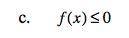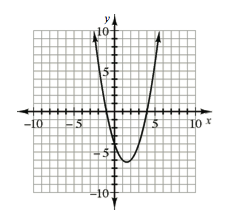Home > CCA2 > Chapter 10 > Lesson 10.2.2 > Problem10-132

10-132.
1.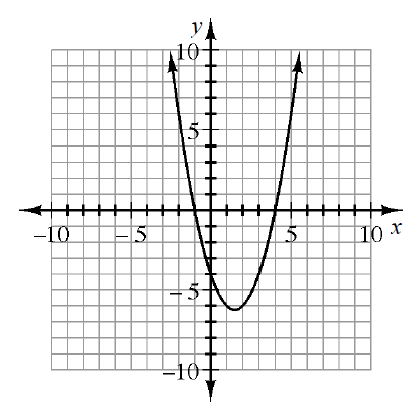The graph of f(x) = x2 − 3x − 4 is shown at right. Use the graph to solve: Homework Help ✎

1. f(x) = 0

2. f(x) ≥ 0

3. f(x) ≤ 0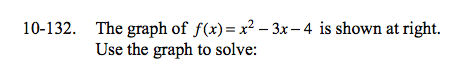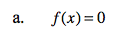Think of f(x) as y.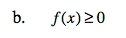x ≤ −1 or x ≥ 4